My Life 时光荏苒，岁月如梭

# Lua 数据类型

2008-02-13

Lua 是动态类型语言，变量不要类型定义,只需要为变量赋值。 值可以存储在变量中，作为参数传递或结果返回。

Lua 中有 8 个基本类型分别为：nil、boolean、number、string、userdata、function、thread 和 table。

nil 这个最简单，只有值 nil 属于该类，表示一个无效值（在条件表达式中相当于 false）。
boolean 包含两个值：false 和 true。
number 表示双精度类型的实浮点数
string 字符串由一对双引号或单引号来表示
function 由 C 或 Lua 编写的函数
userdata 表示任意存储在变量中的 C 数据结构
table Lua 中的表（table）其实是一个”关联数组”（associative arrays），数组的索引可以是数字或者是字符串。在 Lua 里，table 的创建是通过”构造表达式”来完成，最简单构造表达式是{}，用来创建一个空表。

``````print(type("Hello world"))      --> string
print(type(10.4*3))             --> number
print(type(print))              --> function
print(type(type))               --> function
print(type(true))               --> boolean
print(type(nil))                --> nil
print(type(type(X)))            --> string
``````

## nil（空）

nil 类型表示一种没有任何有效值，它只有一个值 – nil，例如打印一个没有赋值的变量，便会输出一个 nil 值：

``````> print(type(a))
nil
>
``````

``````tab1 = { key1 = "val1", key2 = "val2", "val3" }
for k, v in pairs(tab1) do
print(k .. " - " .. v)
end

tab1.key1 = nil
for k, v in pairs(tab1) do
print(k .. " - " .. v)
end
``````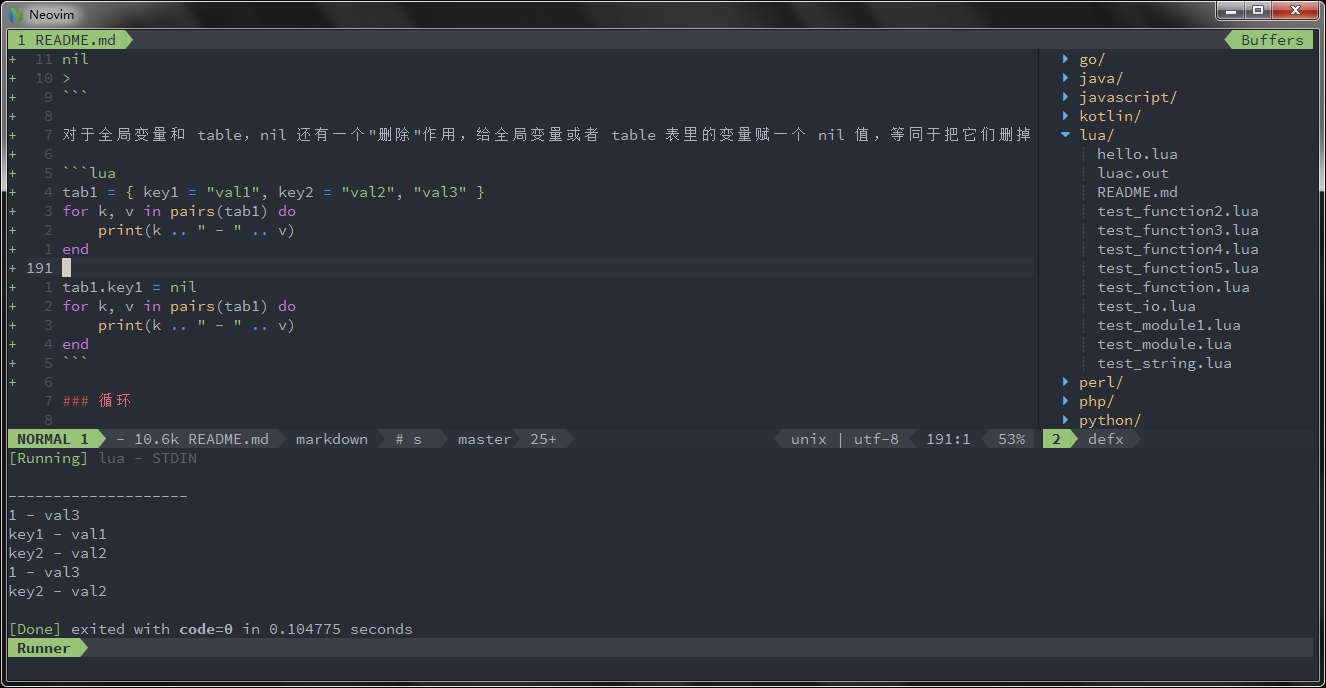## boolean（布尔）

boolean 类型只有两个可选值：true（真） 和 false（假），Lua 把 false 和 nil 看作是”假”，其他的都为”真”:

``````print(type(true))
print(type(false))
print(type(nil))

if type(false) or type(nil) then
print("false and nil are false!")
else
print("other is true!")
end
``````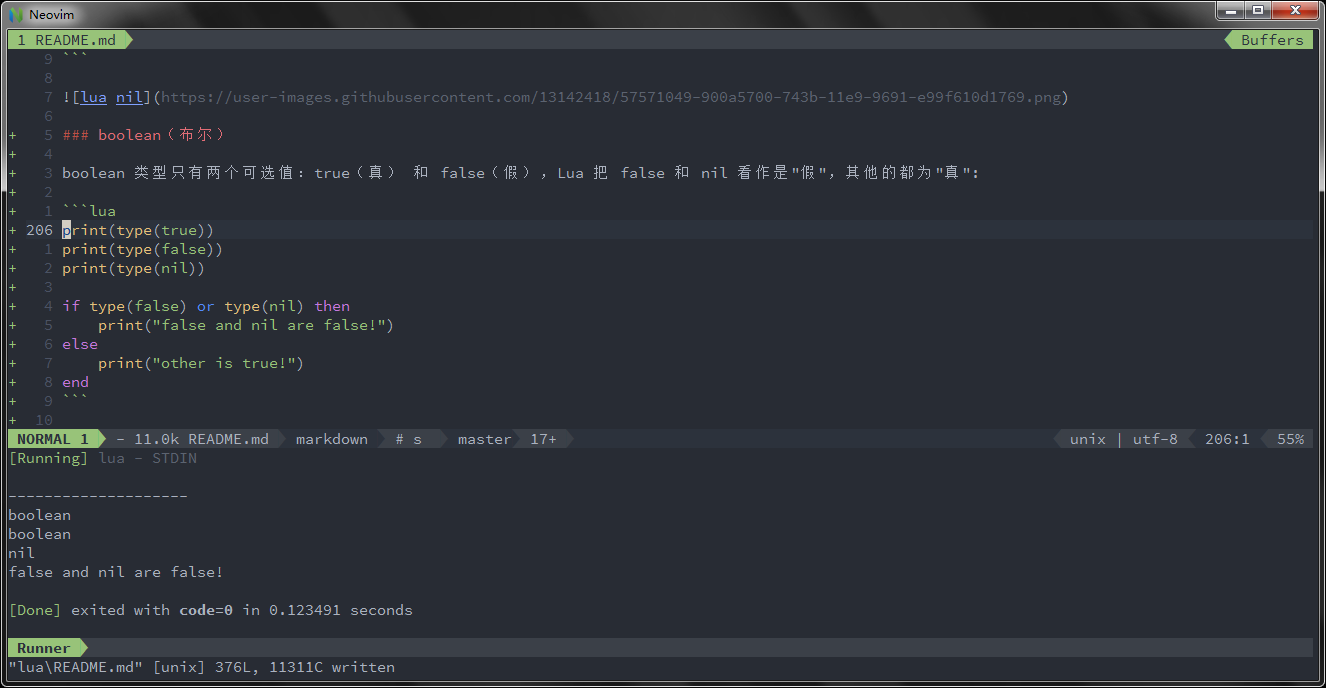## number（数字）

Lua 默认只有一种 number 类型 – double（双精度）类型（默认类型可以修改 luaconf.h 里的定义），以下几种写法都被看作是 number 类型：

``````print(type(2))
print(type(2.2))
print(type(0.2))
print(type(2e+1))
print(type(0.2e-1))
print(type(7.8263692594256e-06))
``````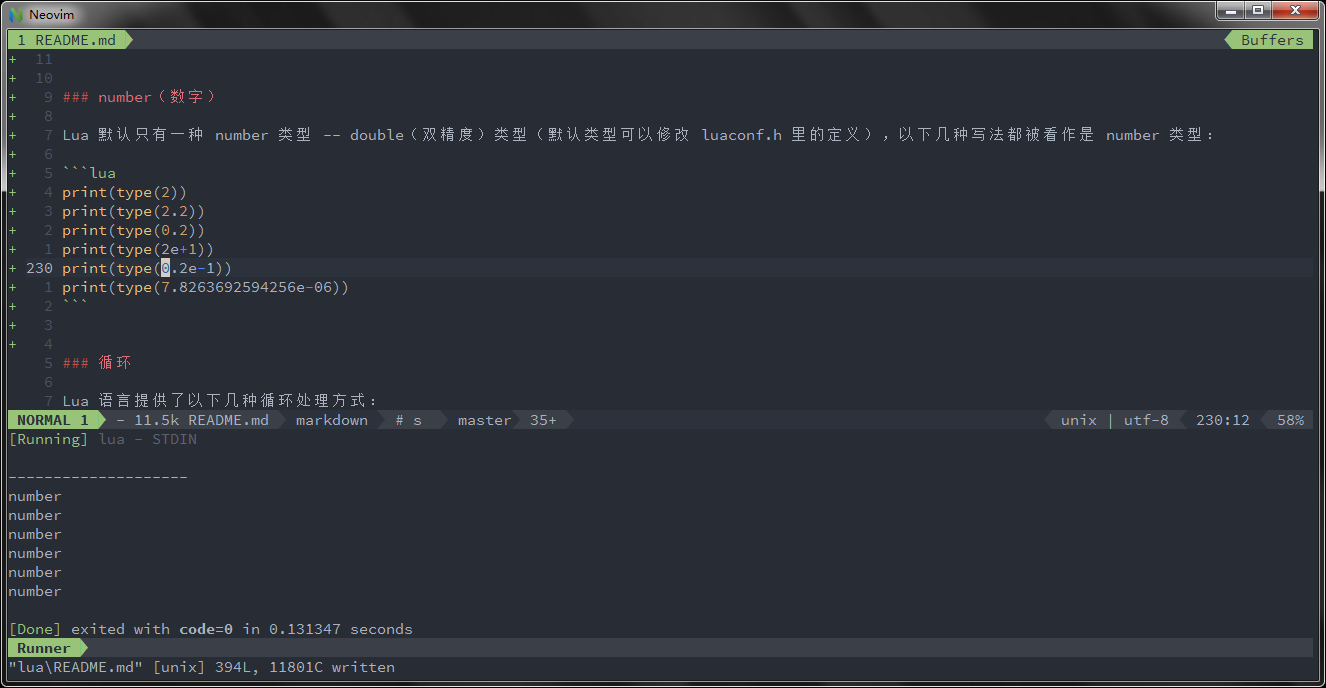## string（字符串）

``````string1 = "this is string1"
string2 = 'this is string2'
``````

``````html = [[
<html>
<body>
<a href="//www.w3cschool.cn/">w3cschoolW3Cschool教程</a>
</body>
</html>
]]
print(html)
``````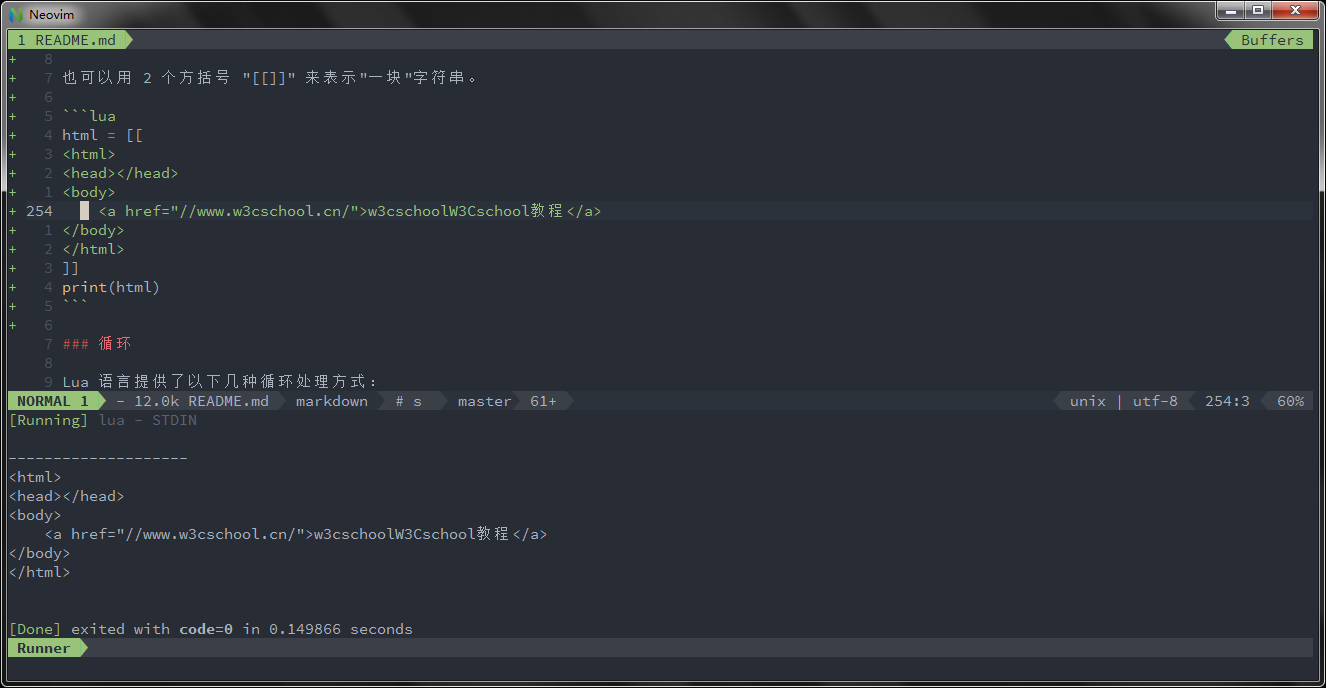``````> print("2" + 6)
8.0
> print("2" + "6")
8.0
> print("2 + 6")
2 + 6
> print("-2e2" * "6")
-1200.0
> print("error" + 1)
stdin:1: attempt to perform arithmetic on a string value
stack traceback:
stdin:1: in main chunk
[C]: in ?
>
``````

``````> print("a" .. 'b')
ab
> print(157 .. 428)
157428
>
``````

``````> len = "www.w3cschool.cn"
> print(#len)
16
> print(#"www.w3cschool.cn")
16
>
``````

## table（表）

``````-- 创建一个空的 table
local tbl1 = {}

-- 直接初始表
local tbl2 = {"apple", "pear", "orange", "grape"}
``````

Lua 中的表（table）其实是一个”关联数组”（associative arrays），数组的索引可以是数字或者是字符串。

``````-- table_test.lua 脚本文件
a = {}
a["key"] = "value"
key = 10
a[key] = 22
a[key] = a[key] + 11
for k, v in pairs(a) do
print(k .. " : " .. v)
end
``````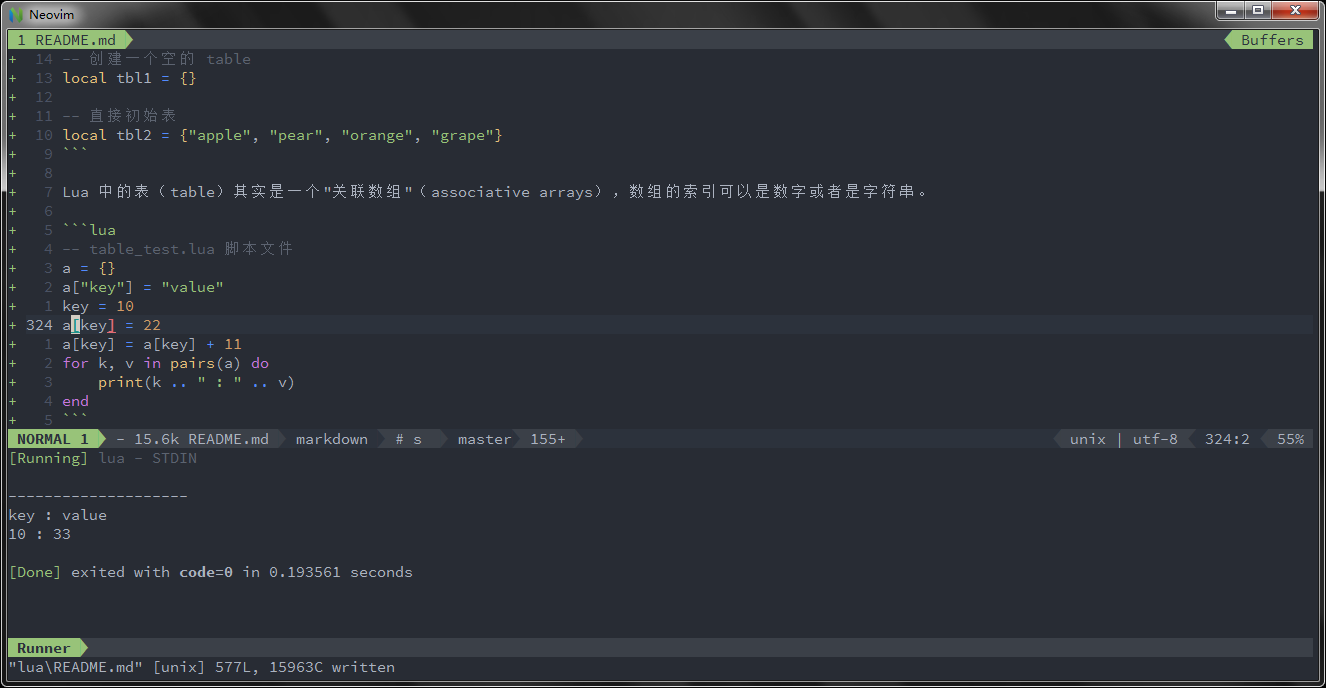``````-- table_test2.lua 脚本文件
local tbl = {"apple", "pear", "orange", "grape"}
for key, val in pairs(tbl) do
print("Key", key)
end
``````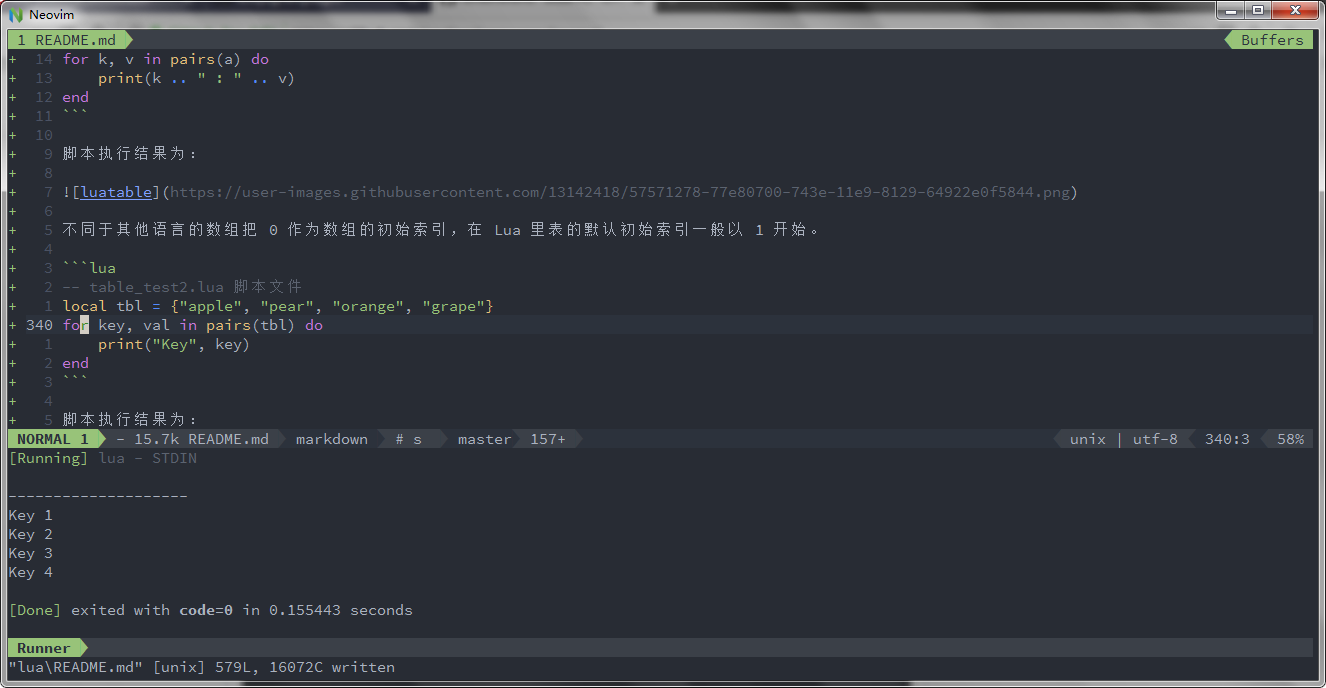table 不会固定长度大小，有新数据添加时 table 长度会自动增长，没初始的 table 都是 nil。

``````-- table_test3.lua 脚本文件
a3 = {}
for i = 1, 10 do
a3[i] = i
end
a3["key"] = "val"
print(a3["key"])
print(a3["none"])
``````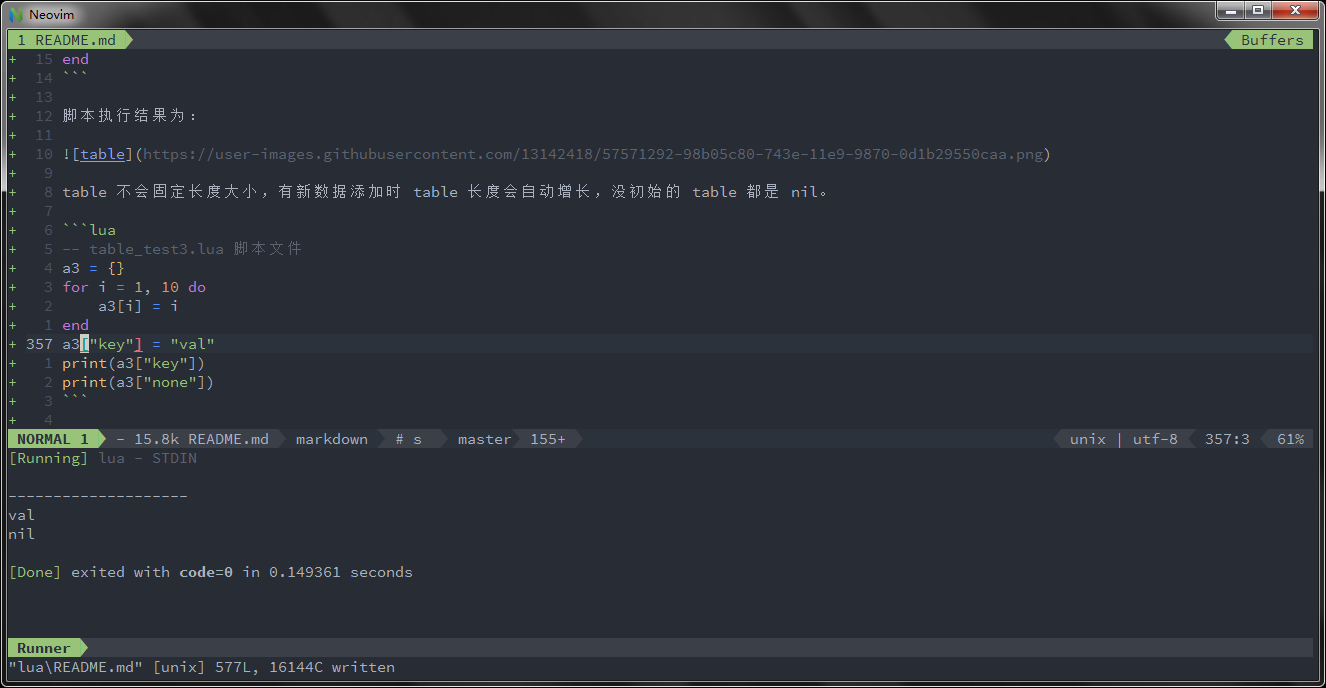## function（函数）

``````-- function_test.lua 脚本文件
function factorial1(n)
if n == 0 then
return 1
else
return n * factorial1(n - 1)
end
end
print(factorial1(5))
factorial2 = factorial1
print(factorial2(5))
``````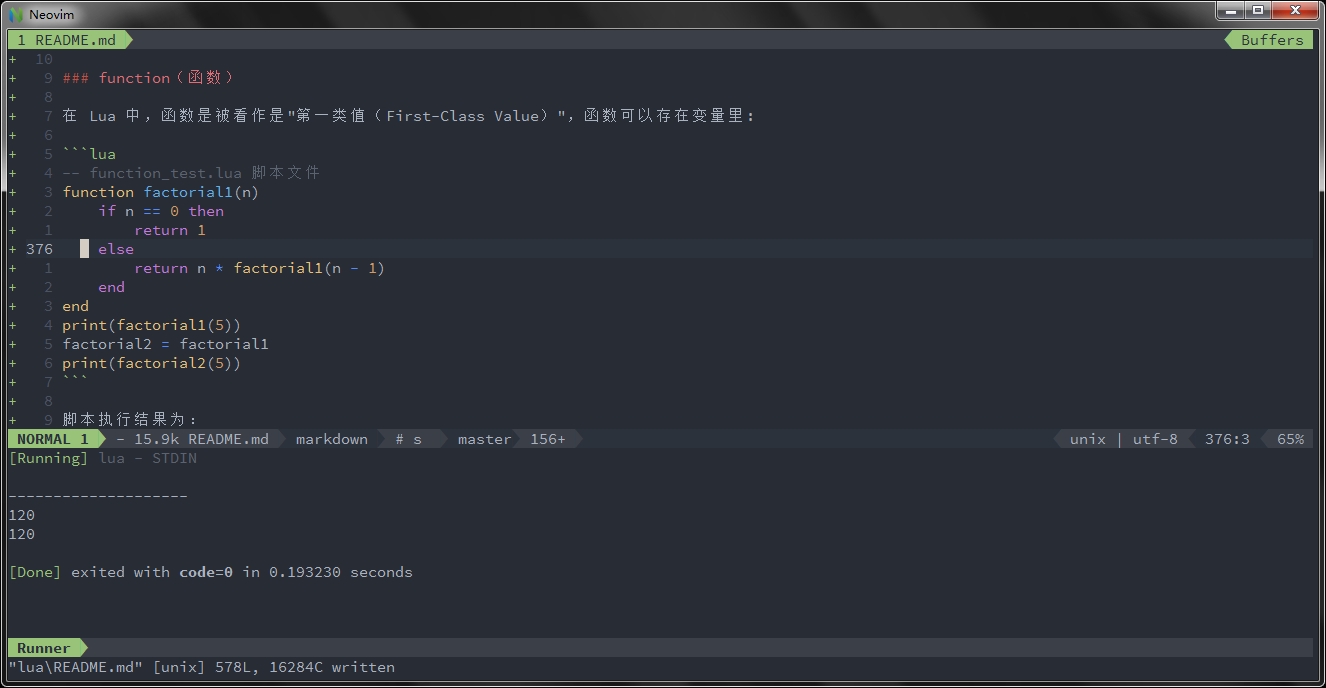function 可以以匿名函数（anonymous function）的方式通过参数传递:

``````-- function_test2.lua 脚本文件
function anonymous(tab, fun)
for k, v in pairs(tab) do
print(fun(k, v))
end
end
tab = { key1 = "val1", key2 = "val2" }
anonymous(tab, function(key, val)
return key .. " = " .. val
end)
``````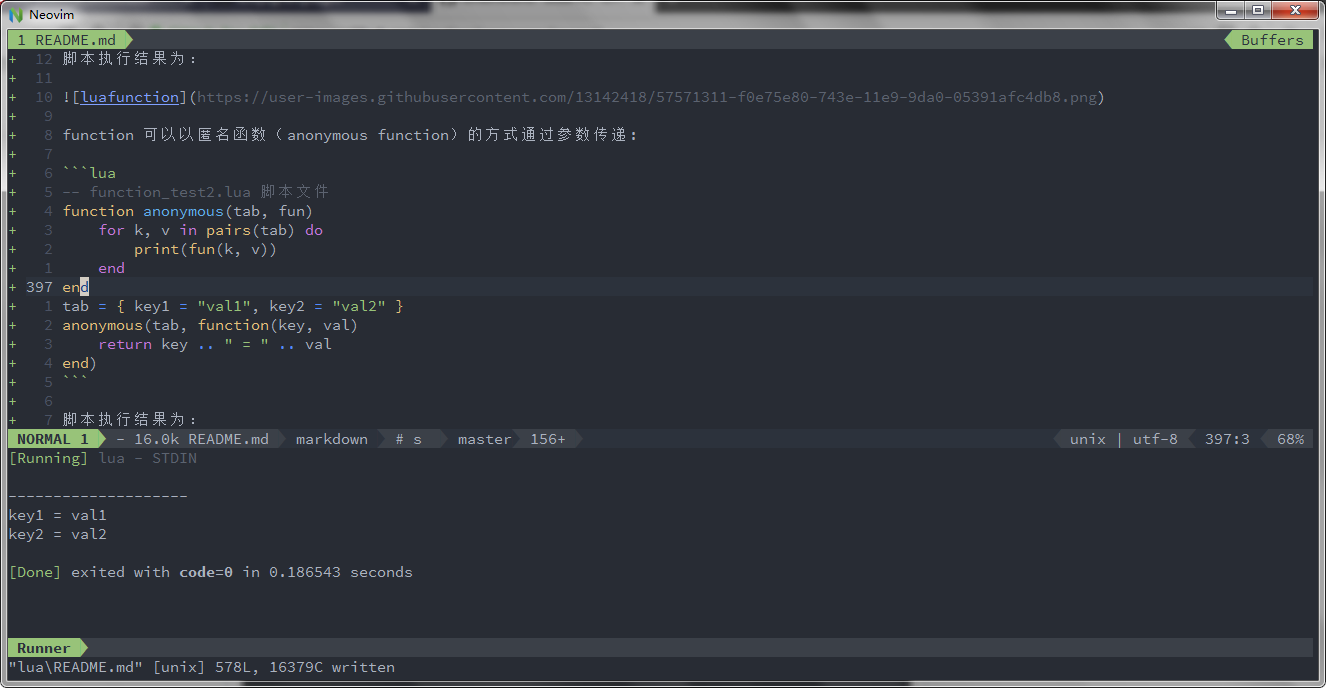## userdata（自定义类型）

userdata 是一种用户自定义数据，用于表示一种由应用程序或 C/C++ 语言库所创建的类型，可以将任意 C/C++ 的任意数据类型的数据（通常是 struct 和 指针）存储到 Lua 变量中调用。

Share on:

## 评论

暂无评论

使用 GitHub issue 参与评论。

目录